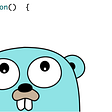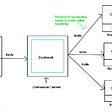# Flipping an Image LeetCode Solution

## Problem Statement

Flipping an Image LeetCode Solution — We are given a matrix of size n. We need to perform 2 tasks-

— flip the image horizontally: it means each row of the given matrix is reversed

— invert the image: make all 0’s to 1’s & vice versa

Return the resulting matrix.

Given matrix contains only 0 or 1 and size of image is ≤ 20.

## Examples & Explanations

Example 1:

Input: image = ,,]

Output: ,,]

Explanation: First reverse each row: ,,].

Then, invert the image: ,,]

Example 2:

Input: image = ,,,]

Output: ,,,]

Explanation: First reverse each row: ,,,].

Then invert the image: ,,,]

## Approach

After reviewing some examples you will notice the following patterns:

1) Look at the first and last value of the row. If they are the same (1,1 or 0,0), they will be flipped in the output.

If they are different (1,0 or 0,1), they do not change. Work your way inward to the middle of the list

applying this rule.

2) If the row has an odd number of entries, the middle value always flips. For example if len(row) = 5,

then row must change values.

Bitwise XOR → 0¹ = 1, 1¹ =0

Let i be the index at the beginning of the row, and j be the index at the end of the row. If the values at

these indices (row and row) are equal, flip their values using XOR ^. If the values are not equal, do

nothing and move i and j closer to the middle. When i == j , the code still executes as it should.

The question has 2 parts-

— Flipping the image: we can simply use another array or vector to store matrix and reverse it for all rows and store the answer in another matrix res

— Inverting the image: this is an easy task, simply check the value of res and change the value

This approach requires us to declare another matrix of size n x n. Can we do better?

We can achieve flipping in place by swapping the elements by pivoting the middle element of every row in the image. For each row, keep the middle element in place and swap the ith element with the (n-1-i)th element. We will use XOR to invert the elements.

We know that 1¹ = 0 & 1⁰ = 1

## Code

C++ code for Flipping an Image

class Solution {

public:

vector flipAndInvertImage(vector& image) {

int n = image.size();

for(auto &row: image) {

for(int i=0; i

--

--

--

## More from leetcode-solutions-tutorialcup

Crack the Coding Interviews

## Functions in Go## A year in perspective## Chef tutorial-1 : Introduction To Configuration Management Tool (CMT) - Chef## Migrate Workloads from OpenShift Templates to Helm Charts: Part 1 of 3## Running Flink Application on Kinesis Data Analytics(KDA)- Part 2## How To Monitor A Flight With An API## Sixth round of Obyte Autonomous Agents Developer Contest## Most Popular Lens Extensions: June 2021## How to get started on Flutter for Mobile App Development## How to perform CRUD Operations in Entity Framework Database First Approach?.## Intro to APIs## Add Translation and Transcription to Voice Calls with SignalWire RELAY